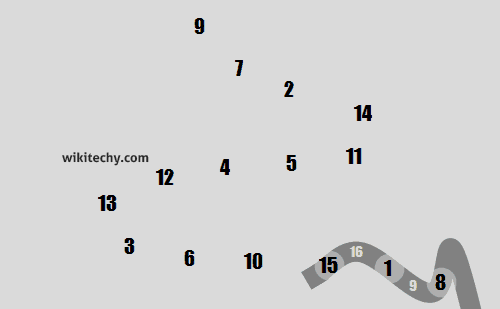# C# Sum - c# - c# tutorial - c# net

## How to write Sum Program in C# ?

• The C# Program Gets a Number and Display the Sum of the Digits. The digit sum of a given integer is the sum of all its digits.
• We can write the sum of digits program in C# language by the help of loop and mathematical operation only.C# Sum

## Sum of digits algorithm

• To get sum of each digit by C# program, use the following algorithm:
• Step 1: Get number by user
• Step 2: Get the modulus/remainder of the number
• Step 3: sum the remainder of the number
• Step 4: Divide the number by 10
• Step 5: Repeat the step 2 while number is greater than 0.
• Let's see the sum of digits program in C#